• matlab机器学习 无约束非线性优化工具包 亲测有效 强大实用 提高效率缩短开发周期 非常好用 强烈推荐
• 线性规划 求解下列线性规划 1 代码 结果 x = (0.3333,1.5000)T,fval= -4.0087 ...无约束非线性优化 求解下列无约束非线性规划问题 代码 结果 fminunc函数结果：x= 1.0e-23 * (0.0827, 0.3309)T,fval= 1.8545e-4
线性规划
求解下列线性规划
1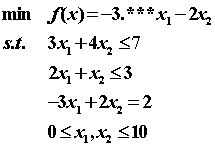代码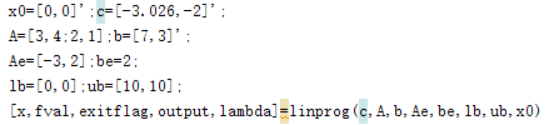结果
x = (0.3333,1.5000)T,fval= -4.0087
2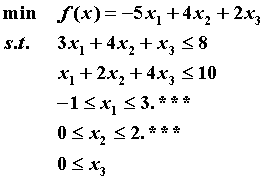代码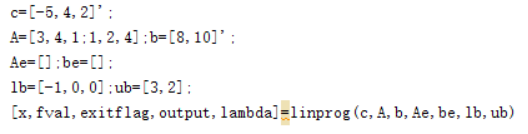结果
x=( 2.6667,0,0)T,fval= -13.3333
二次规划
求解下列二次规划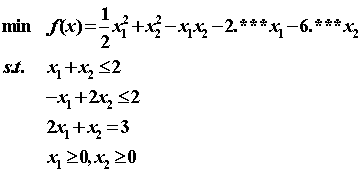代码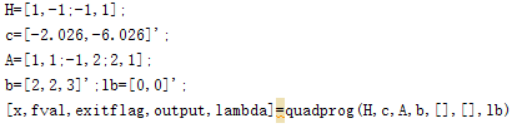结果
x=( 0.6667, 1.3333)T,fval= -9.1631
无约束非线性优化
求解下列无约束非线性规划问题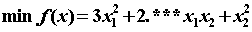代码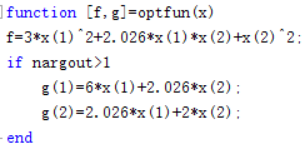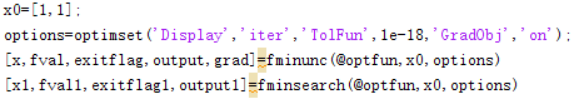结果
fminunc函数结果：x= 1.0e-23 * (0.0827, 0.3309)T,fval= 1.8545e-47。
fminsearch函数结果：x1= 1.0e-09*(0.4642, -0.0830)T,fval= 5.7538e-19。
约束非线性规划问题
求解下列约束非线性规划问题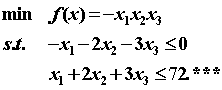代码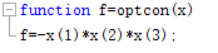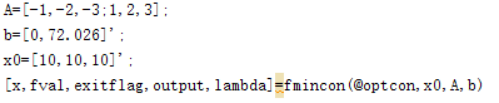结果
x=( 24.0087,12.0043,8.0029)T,fval= -2.3065e+03。


展开全文matlab 动态规划
• 该函数展示了一种使用扩展卡尔曼滤波器解决一些无约束非线性优化问题的方法。 包括两个示例：一般优化问题和求解由神经网络模型表示的一组非线性方程的问题。 该函数需要扩展卡尔曼滤波函数，可从以下链接下载： ...matlab
• 采用无约束非线性优化算法对反演过程中相关曲线的拟合问题进行了分析和研究, 实现了从任意初始值开始获取最优非线性拟合解, 并给出了算法的实现步骤。采用此方法对50 nm, 100 nm, 200 nm和500 nm的标准聚苯乙烯乳胶...
• 结合LS方法与CD方法给出混合的共轭参数和相应的谱参数,建立采用标准Wolfe线搜索的谱共轭梯度算法,证明了算法满足下降性和全局收敛性,数值试验显示算法是有效的,适合于求解大型无约束非线性优化问题.研究结果表明:谱...
• 文章目录无约束非线性优化线搜索方法搜索方向步长例子 无约束非线性优化 Unconstrained Nonlinear Programming 一个典型的UNP问题可以写为： minimize f(x) \text{minimize} \ f(x) minimize f(x) 线...
文章目录无约束非线性优化通用下降方法下降方向步长例子
无约束非线性优化

Unconstrained Nonlinear Programming

一个典型的UNP问题可以写为：
$\text{minimize} \ f(x)$
通用下降方法

General Descent Method

是一种用迭代的方式求目标函数最小值的方法，每次迭代都会计算搜索方向和步长
$x_{k+1} = x_k + t_k p_k$
其中，$p$ 是搜索方向， $t$ 是步长
之所叫下降方法，是因为满足 $f\left(x_{k+1}\right)
下降方向

Descent Direction

引入梯度（Gradient）的概念，用 $\nabla$ 来表示，梯度默认指正梯度，这里下降使用的是负梯度，即 $-\nabla f(x)$ 。只要搜索方向和负梯度方向的夹角小于90°，且步长合适的情况下，都是下降方向，表示为：$\nabla f\left(x_{k}\right)^{T} p_{k}<0$ 。
搜索方向有个一般式： $p_k=−B_k^{−1}\nabla f(x_k)$
其中 $B_k$ 是一个对称非奇异矩阵，有以下几种常见的下降方法

梯度下降法（也叫最陡下降法）（Steepest Descent）
$B_k$ 是单位阵，即直接选负梯度为搜索方向，表示为：$p_{k}=-\nabla f\left(x_{k}\right)$

牛顿方法（Newton）
$B_k = ∇^2f(x_k)$ 是海森（Hessian）矩阵

拟牛顿法

共轭梯度法

补充一点，我们将一般式两边同乘，得到 $\nabla f\left(x_{k}\right)^{T} p_k=−\nabla f\left(x_{k}\right)^{T} B_k^{−1}\nabla f(x_k)$，当 $B_k$ 是正定矩阵时，$B_k^{-1}$ 也是正定的，因此根据正定的性质，这个式子是满足 $\nabla f\left(x_{k}\right)^{T} p_k < 0$ 的，印证了下降方向。
步长

Stepsize

很多时候我们用的步长是一个固定值，但在这里，我们使用线搜索（Line Search）的方法，每一次迭代都会重新计算步长，有两种方式：

精确线搜索（Exact Line Search）
求解下面这个优化问题，其实很容易理解。步长如果太小，则下降不够快；步长如果太大，则可能不降反升，因此我们想找到步长的一个上界。
$t_k = \underset{\alpha \geq 0}{\arg\min} f(x_k + \alpha p_k)$

回溯线搜索（Backtracking Line Search）
上面精确计算有时候会很复杂，其实只要近似估计就可以了，设想是先初始化一个较大的步长，然后逐渐缩小，以使得新的函数值与旧的函数值的减少程度大于预设的期望值。可以考虑步长从单位1开始。
如果 $f(x_k+t_k p_k)>f(x_k)+\alpha t_k \nabla f(x_k)^{T} p_k$ ，则令 $t_{k+1} = \beta t_k$
其中 $\alpha \in(0,0.5)$   $\beta \in(0,1)$   $t_0 = 1$
$f(x_k+t_k p_k) \leq f(x_k)+\alpha t_k \nabla f(x_k)^{T} p_k$ 被称为Armijo准则，和 $\nabla f\left(x_{k}+t_{k} p_{k}\right)^{T} p_{k} \geq \alpha_{2} \nabla f(x_k)^{T} p_{k}$ 一起构成了Wolfe条件

补充：之所以叫线搜索是因为选定的步长 $t$ 将决定从直线 {$x+tp$} 上哪一点开始下一步迭代，其实更准确的应该被叫做射线搜索（Ray Search）
需要知道Armijo准则是一定能满足的，因为只要步长足够小，就一定有
$f(x+t \Delta x) \approx f(x)+t \nabla f(x)^{T} \Delta x
例子
用梯度下降和精确先搜索方法求解如下问题：
$\text { minimize } f(x)=\frac{1}{2}\left(x_{1}^{2}+\gamma x_{2}^{2}\right)$

确定搜索方向，这里直接用负梯度：
$p_k = (-x_{1(k)}, -\gamma x_{2(k)})^T$

确定步长：
$t_k = \underset{\alpha \geq 0}{\arg\min} \frac{1}{2}\left[(1-\alpha)^{2} x_{1(k)}^{2}+\gamma(1-\alpha \gamma)^{2} x_{2(k)}^{2}\right]$
可以直接用配方法得到：
$t_k = \frac{x_{1(k)}^2 + \gamma^2 x_{2(k)}^2}{x_{1(k)}^2 + \gamma^3 x_{2(k)}^2}$

应用线搜索方法：
$x_{k+1} = x_k + \frac{x_{1(k)}^2 + \gamma^2 x_{2(k)}^2}{x_{1(k)}^2 + \gamma^3 x_{2(k)}^2} (-x_{1(k)}, -\gamma x_{2(k)})^T$
将 $x_1, x_2$ 分成两项即：
$x_{1(k+1)} = x_{1(k)} - \frac{x_{1(k)}^2 + \gamma^2 x_{2(k)}^2}{x_{1(k)}^2 + \gamma^3 x_{2(k)}^2} x_{1(k)} = \frac{(\gamma - 1)\gamma^2 x_{2(k)}^2}{x_{1(k)}^2 + \gamma^3 x_{2(k)}^2} x_{1(k)}$
$x_{2(k+1)} = x_{2(k)} - \frac{x_{1(k)}^2 + \gamma^2 x_{2(k)}^2}{x_{1(k)}^2 + \gamma^3 x_{2(k)}^2} \gamma x_{2(k)} = \frac{(1 - \gamma) x_{1(k)}^2}{x_{1(k)}^2 + \gamma^3 x_{2(k)}^2} x_{2(k)}$

选择一个初始点开始迭代，这里选一个较特殊的点 $x_{1(0)} = \gamma$，$x_{2(0)} = 1$
当 $k =0$ 时，有：
$x_{1(1)} = \gamma\left(\frac{\gamma-1}{\gamma+1}\right)$
$x_{2(1)} = -\left(\frac{\gamma-1}{\gamma+1}\right)$
当 $k =1$ 时，有：
$x_{1(2)} = \gamma\left(\frac{\gamma-1}{\gamma+1}\right)^2$
$x_{2(2)} = -\left(\frac{\gamma-1}{\gamma+1}\right)^2$
通过数学归纳法，我们可以得到一个通式：
$x_{1(k)} = \gamma\left(\frac{\gamma-1}{\gamma+1}\right)^k$
$x_{2(k)} = -\left(\frac{\gamma-1}{\gamma+1}\right)^k$


展开全文• 本博客仅为学习笔记。梯度下降法有函数x(θ)x(\theta) ,梯度下降法的迭代公式为: xk+1=xk−agk x_{k+1} = x_k -a g_k 其中gkg_k为x(θ)x(\theta) 在x_k点的导数。牛顿法当x为标量时 xk+1=xk−x′x′′ ...
本博客仅为学习笔记。

梯度下降法

有函数x(θ)<!--//--><![CDATA[//><!--
x(\theta)
//--><!]]> ,梯度下降法的迭代公式为:

xk+1=xk−agk<!--//--><![CDATA[//><!--
x_{k+1} = x_k -a g_k
//--><!]]>
其中gk<!--//--><![CDATA[//><!--
g_k
//--><!]]>为x(θ)<!--//--><![CDATA[//><!--
x(\theta)
//--><!]]> 在x_k点的导数。

牛顿法

当x为标量时

xk+1=xk−x′x′′<!--//--><![CDATA[//><!--
x_{k+1} = x_k - \frac{x'}{x''}
//--><!]]>当x为向量时： xk+1=xk−H−1kgk<!--//--><![CDATA[//><!--
x_{k+1}  = x_k - H_k^{-1}g_k
//--><!]]>其中 H−1<!--//--><![CDATA[//><!--
H^{-1}
//--><!]]> 为 Hession矩阵的逆函数，g为一阶导数向量。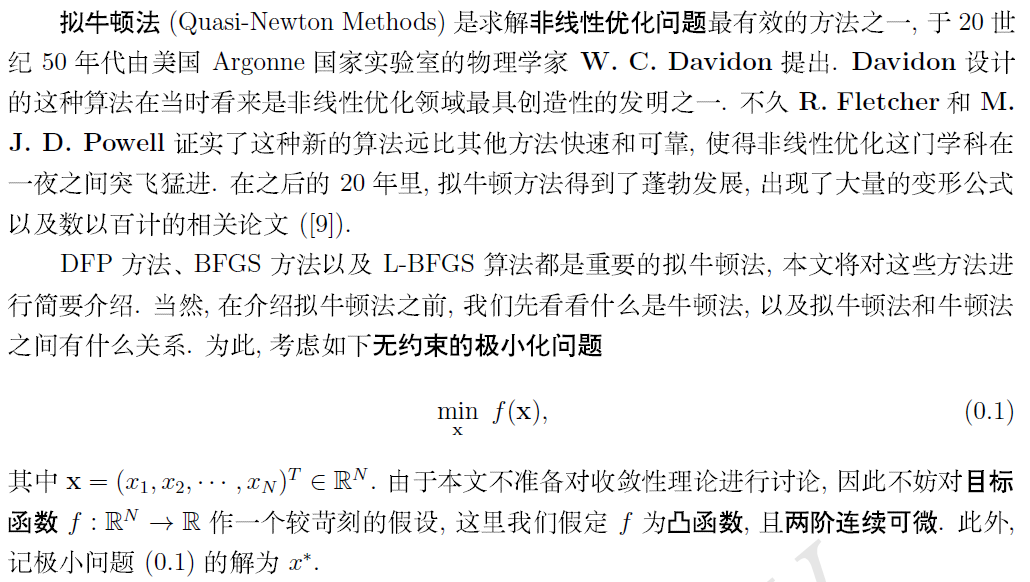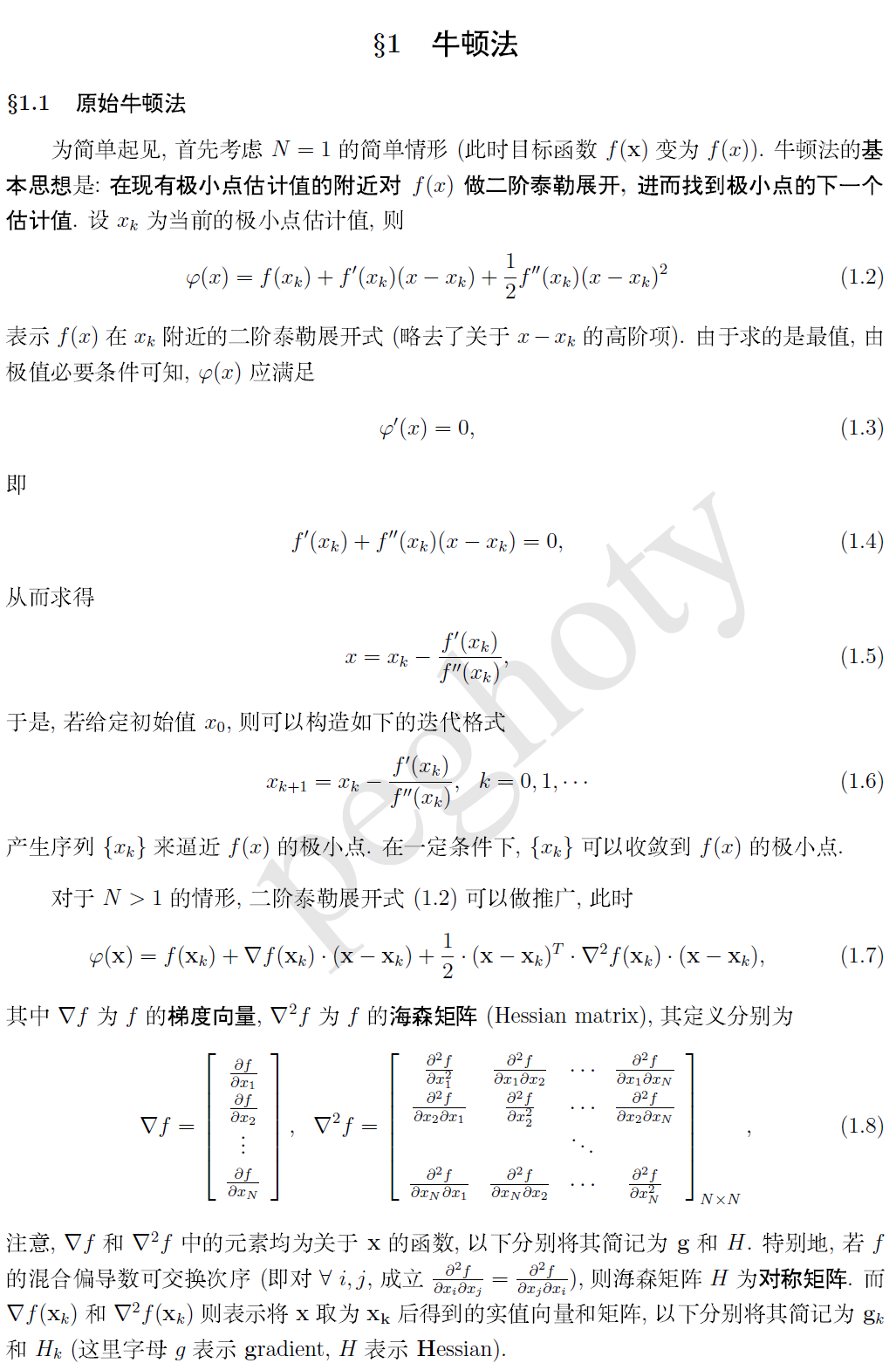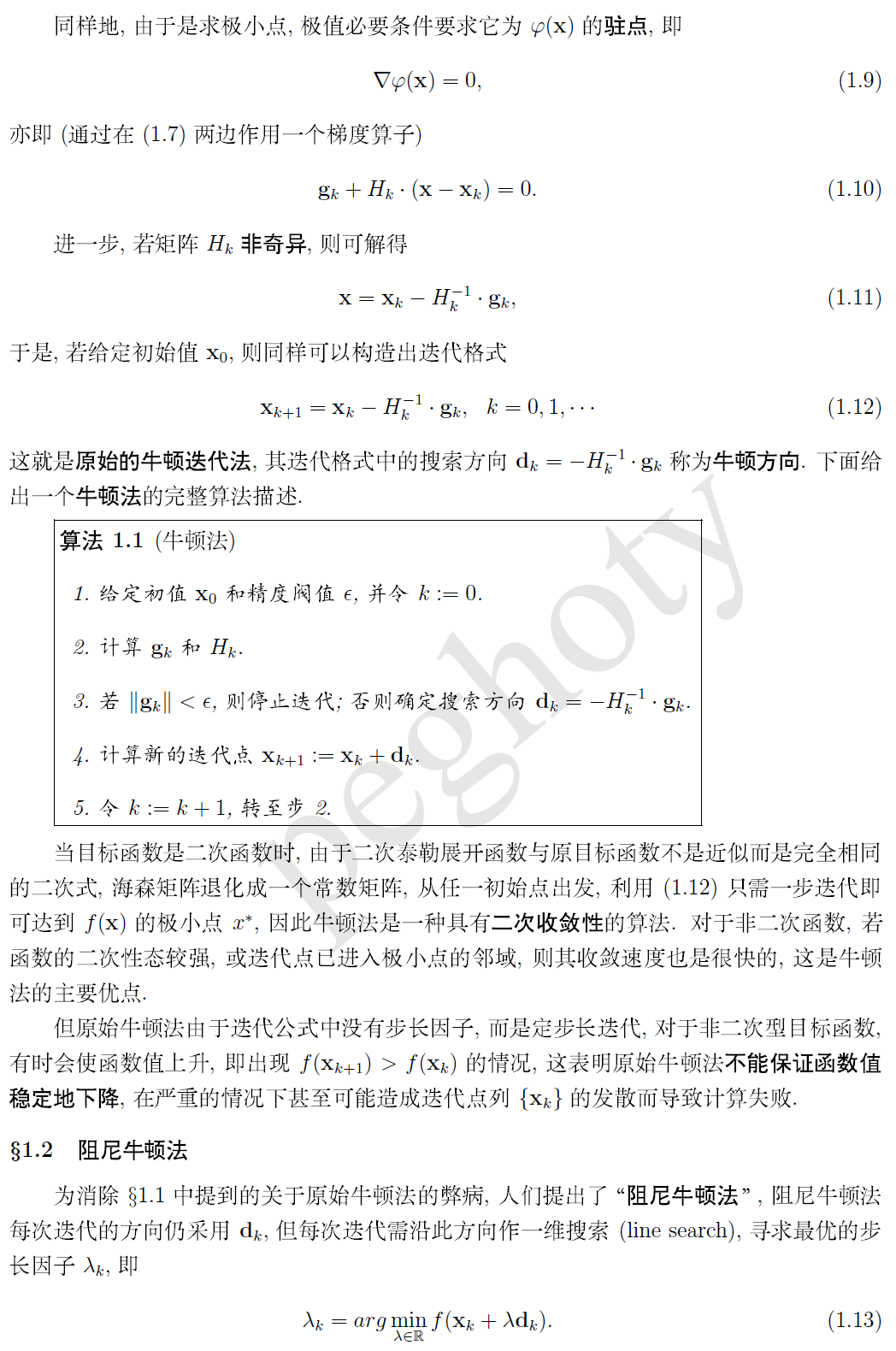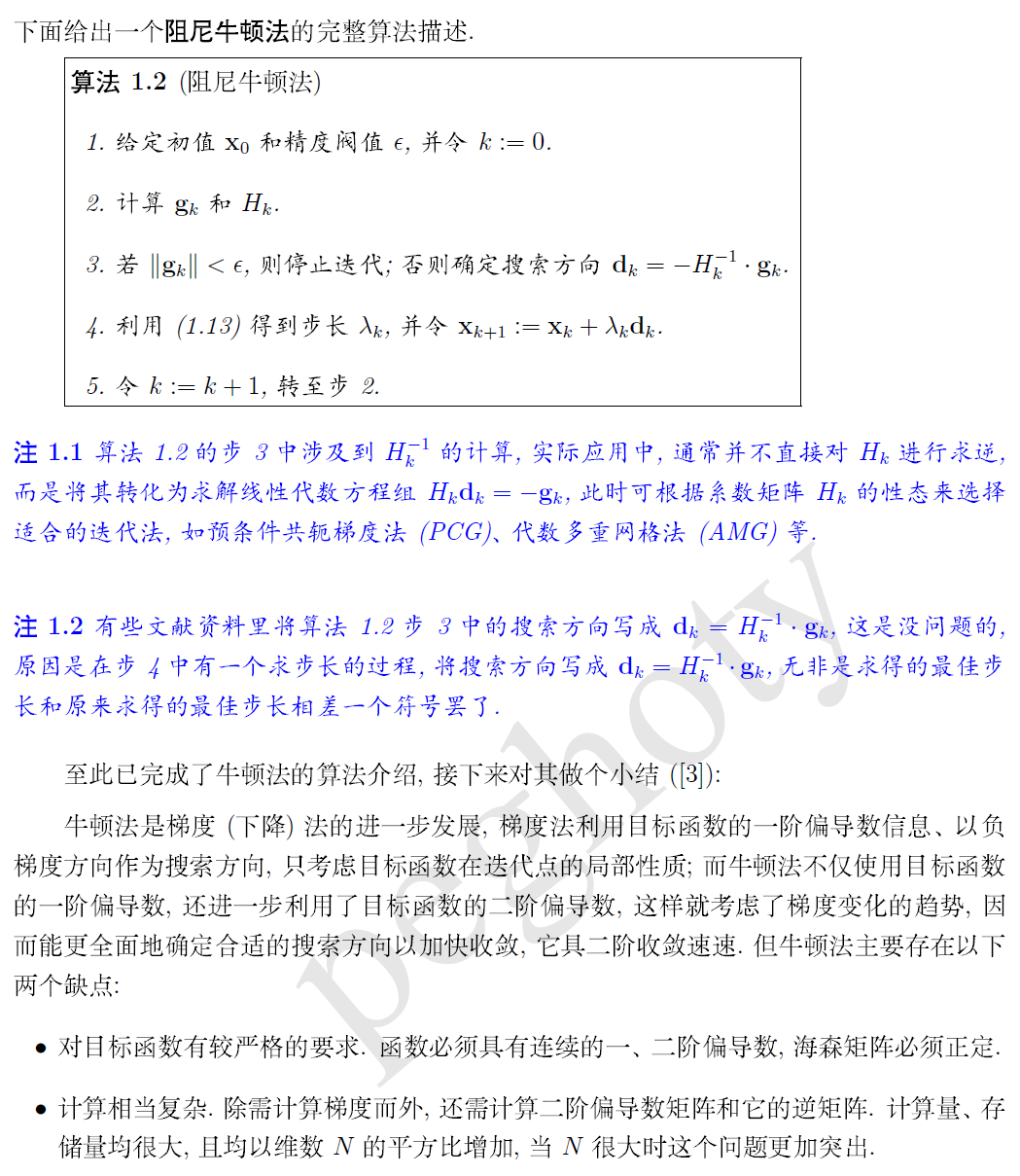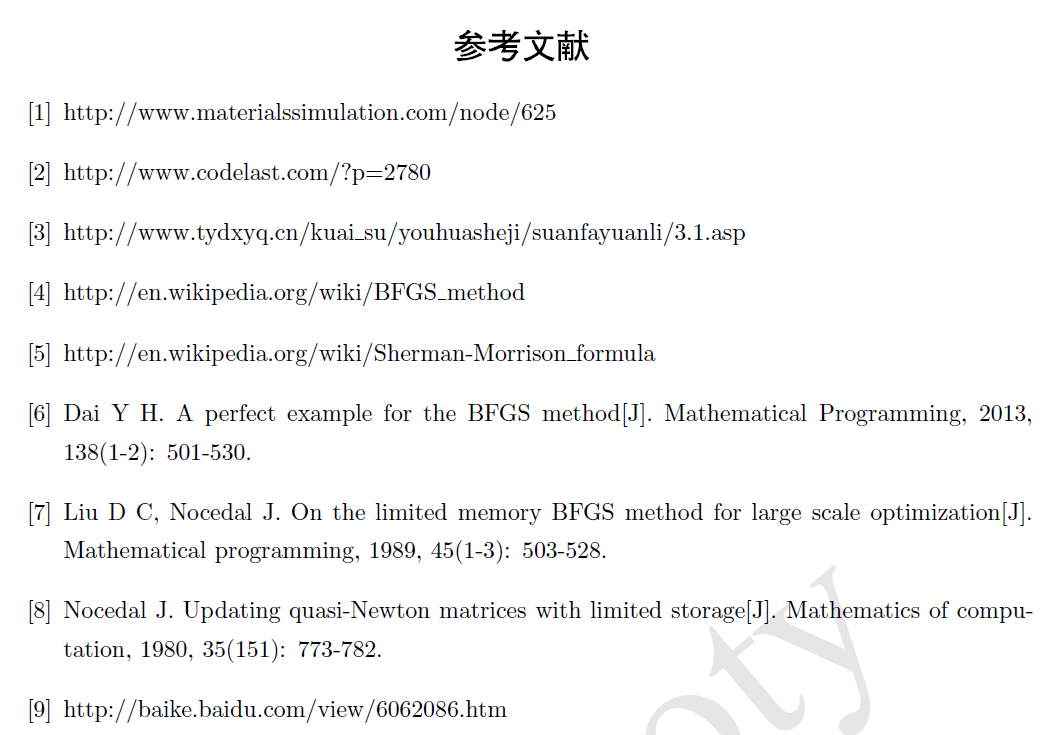L-BFS

在牛顿法中，每次都需要求H−1k<!--//--><![CDATA[//><!--
H_k^{-1}
//--><!]]>, 每次求二阶导数，再求逆矩阵这个计算量是很大的。所以有人考虑使用迭代计算的方法计算每一个H−1k<!--//--><![CDATA[//><!--
H_k^{-1}
//--><!]]>, 令 Bk=H−1k<!--//--><![CDATA[//><!--
B_k = H_k^{-1}
//--><!]]>找出一个公式 ,使得 Bk+1=Bk+δ<!--//--><![CDATA[//><!--
B_{k+1} =B_k + \delta
//--><!]]>
求出这个迭代用的δ<!--//--><![CDATA[//><!--
\delta
//--><!]]>。

http://blog.csdn.net/acdreamers/article/details/44728041
展开全文• 计算机视觉算法和机器学习算法大量使用了最优化理论。这篇文章简单总(fan)结(yi)了...包括了数值优化的定义，分类，以及无约束非线性优化算法概述，介绍了梯度下降法，牛顿法，拟牛顿法，BFGS, LM等几个经典算法。
计算机视觉算法和机器学习算法大量使用了最优化理论。 这篇文章简单总(fan)结(yi)了一下数值优化这本书的第一章到第四章。包括了数值优化的定义，分类，以及无约束的非线性优化算法概述，介绍了梯度下降法，牛顿法，拟牛顿法，BFGS, LM等几个经典算法。

主要参考文献：

 Numerical Optimization. Nocedal, Jorge, Wright, S.
 Computer vision: models, learning and inference. Simon J.D. Prince
 最优化理论与方法, 袁亚湘

数值优化

数值优化指的是在一定的条件下，通过选择变量，最小化目标函数。

写成数学语言，

且,

其中，

参数向量
目标函数
等式约束
不等式约束
中举了一个具体的例子，

假设目前需要优化以下函数，

s.t.

套一下上面的数学符号，

目标函数，

,

参数向量，

,

约束条件，

下图为该问题的几何表示，图中， 为最优解，c1,c2分别代表了两个不等式约束，圆形虚线为的等高线。白色区域为可行域(feasible region)，即满足所有约束的点集。灰色区域为不可行域(infeasible region)。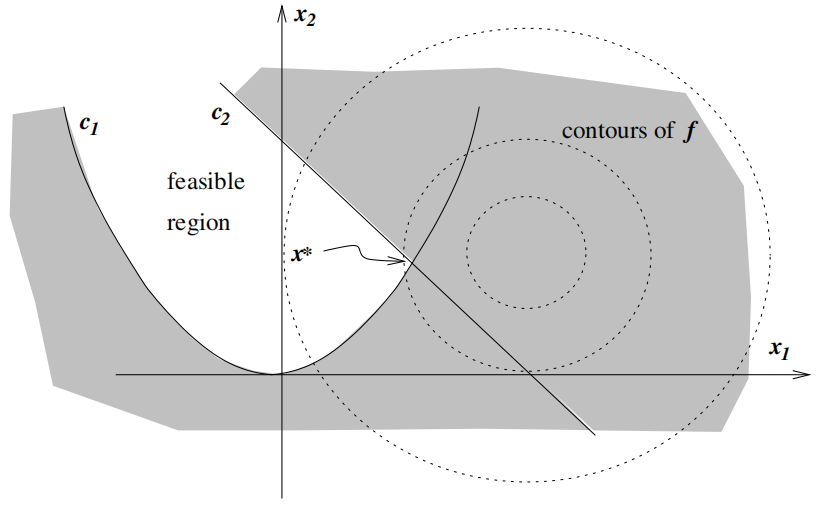分类

数值优化问题包含了很多不同角度的分类。在中的第一章有着详细的说明，挑几个列在下面。

线性与非线性

按照目标函数和约束条件的线性非线性可分为，

线性规划问题（Linear programming problem)。目标函数与约束都是线性函数。
非线性规划问题（non-linear programming problem)。目标函数或约束条件是非线性函数。
连续与离散

根据目标函数的连续与离散，可分为，

离散优化（Discrete Optimization）。离散优化的可行集(Feasible set)是有限的，通常也是非凸的，例如组合规划问题，整数规划问题。
连续优化（Continous Optimization）。由于目标函数由连续的，因此其可行集(feasible set)是无限。这类问题通过求导较容易求解。
离散问题也可以重新定义成一组连续优化的问题来求解。
约束和无约束

根据约束条件不同可以分为有约束问题和无约束问题。显然，无约束问题通常容易求解。有约束的问题也可以转换成一组无约束的问题优化。

凸与非凸

根据目标函数凸，非凸的不同，可以分为凸优化，非凸优化

凸函数和非凸函数的区别如下图所示，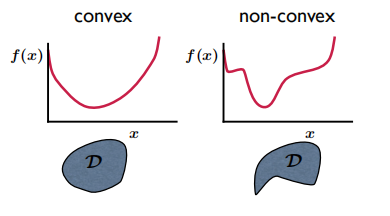凸函数(Convex)定义，

从上图可以看到，对于凸函数来说，局部最优就是全局最优，因此采用局部优化算法就可以实现全局优化。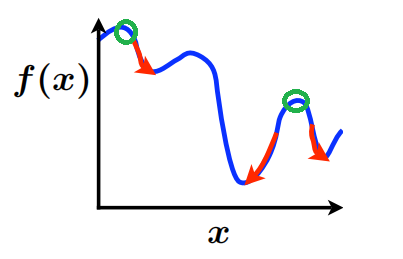局部最优算法也是非凸函数全局最优算法的一种解决方法，比如说，通过多个初始点，从每个初始点开始做局部优化算法，运气好的话，同样可以达到全局优化的目的。

全局与局部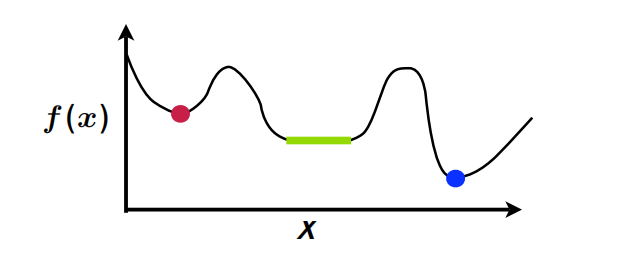对于凸函数来说，局部极值就是全局极值。而非凸函数二者还是有明显的不同。上图描述了优化算法中几种极值点。

全局极小点(Global minimum):  。
严格局部极小点(Strong local minimum): ，定义N为的邻居集合。
局部极小点(Week local minimum): 。
无约束非线性优化

无约束优化问题不需要考虑约束条件。通常还需要假设目标函数足够平滑（二阶可微）。这种算法通常分为基于梯度的算法与导数无关算法。显然，基于梯度的算法更趋向于求解局部最优，而导数无关的算法通常可以求解全局最优。

图像处理和机器学习算法采用了大量基于梯度的最优化算法，这类算法通常采用迭代的方式求解，即，给定一个初始点x0，按照某一种迭代规则生成一个个迭代点序列{xk}，当该序列为有穷点时，最后一个点为模型最优解，当其为无穷点时，其极限为最优解。

基于梯度的算法算法框架如下，

Choose initial variable x0, k=0
while (not converged)
//choose direction p_k and step length α_k
[p_k, α_k] = compute()
x_k+1 = x_k + α_k p_k
k++
end

迭代函数为 , 定义了下降方向，定义了步长。

这类方法又可以分为两大类，线性搜索算法和信赖域算法。

线性搜索(Line search)首先计算方向p_k，然后通过计算 求出α。
信赖域(Trust region)首先选择一个，使得在范围内（信赖域）。目标函数用n为二次模型来逼近，以此选择一个搜索方向sk, 取xk+1 = xk+sk。
梯度下降法(Steepest descent)

梯度下降法属于线性搜索算法的一种，定义梯度方向为下降方向，

然后确定。此时梯度下降法通常不会真正通过优化函数求。而是设定一个最大迭代数与固定步长（或者可变步长 ），在迭代过程中检查收敛性，不停的逼近最优解。

吴恩达机器学习课程里的可变步长的一个例子：

步长随迭代次数下降。

梯度下降法的每一次迭代考虑的是这一步的最快下降方向，也可说他反映的是一种局部性质。而某些情况下，当前最优的方向未必是全局最优的方向。因此，梯度下降在很多情况下会出现锯齿现象，影响了收敛速度。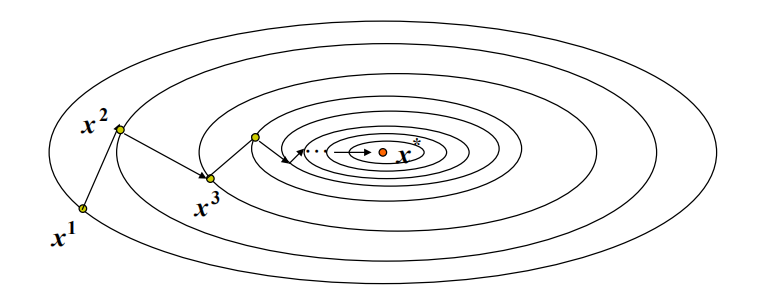牛顿法(Newton Method)

牛顿法在梯度下降法的基础上，增加了二阶导数，改善了上述问题。二阶导数意味着梯度的变化。也就是说牛顿法不仅考虑了梯度本身还考虑了梯度的变化。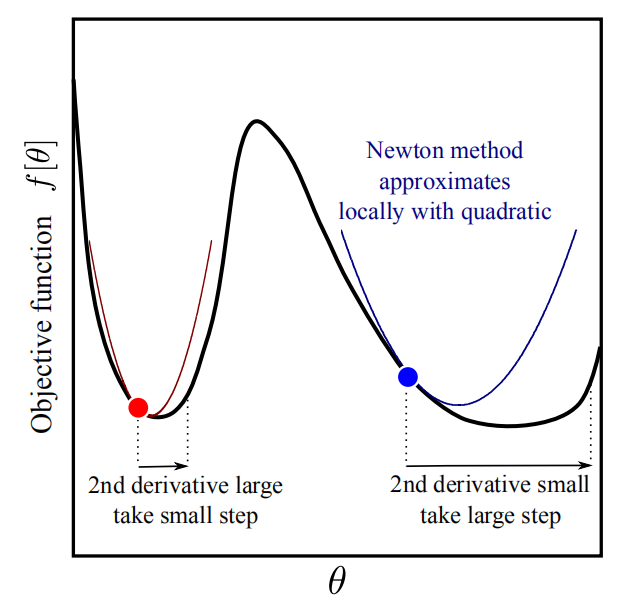如上图所示，红色点和蓝色点的梯度(一阶导数)相同，但是二阶导数不同。红点二阶导数大于蓝色点。也就是说红点的梯度变化更大。如果梯度变化快，那么移动的距离就要小一点。同样的，如果梯度变化慢，那么移动的距离就可以大一点。

将目标函数二阶展开，即可同时考虑梯度和梯度变化。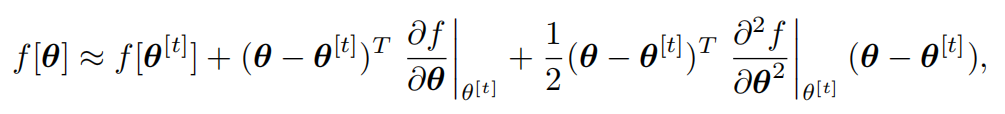为求 的极值，等式两边对求导，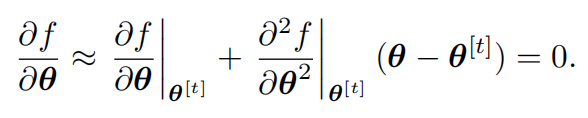得到使最小的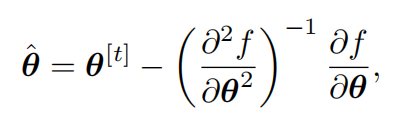结合梯度法的迭代函数 ，我们把牛顿法的迭代公式写作，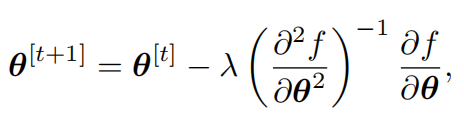因此，下降方向为，

为步长。通常牛顿法的步长可以设置为1。特别的，如果目标函数是一个二次函数，那么可以直接一步迭代得到最优解。

中给出了一组梯度下降法和牛顿法的比较。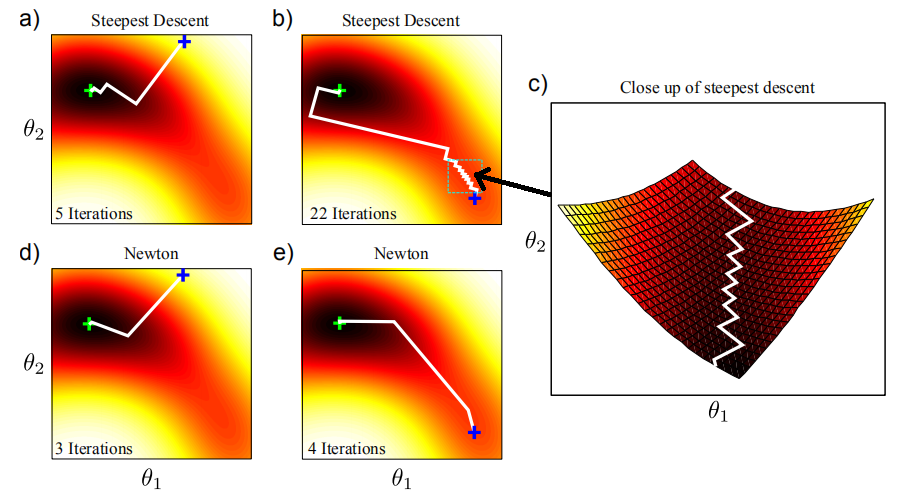目标函数从蓝叉优化到绿叉。图a,和b采用梯度下降法，d和e采用牛顿法。图c为图b锯齿区域的放大示意。可以看到牛顿法的迭代次数比梯度下降法少很多。

然而，牛顿法采用了的二阶导数的逆矩阵，如果Hessian矩阵不是正定矩阵，那么牛顿方向不一定是目标函数的下降方向。

由于目标函数在极点的Hessian矩阵是正定的，那么在他附近的点的Hessian矩阵也可以认为是正定的，因此，当初始点在极点附近时，牛顿法有效且收敛速度较快。

拟牛顿(Quasi-Newton)法

拟牛顿法构造了一个对称正定矩阵 来近似Hessian矩阵，在牛顿法的基础上进一步完善了优化算法。拟牛顿法的下降方向可记为，

拟牛顿法算法比牛顿法增加了一行，更新，。初始化为单位矩阵。这样每次更新不需要矩阵求逆。

Choose initial variable x0, k=0，H_0=I
while (not converged)
p_k = -H_k g_k
x_k+1 = x_k + α_k p_k
H_k+1 = update(H_k)
k++
end

常见的，更新公式，

对称秩一校正公式(symmetric-rank-one)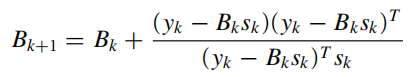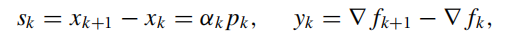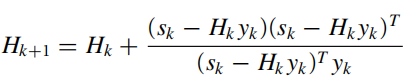BFGS校正公式(Broyden, Fletcher, Goldfarb, and Shanno)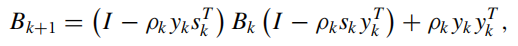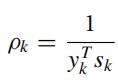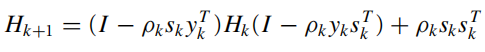信赖域(Trust region)法

与上面的几个方法不同，信赖域法第一步不去计算搜索方向，而是首先选择一个长度，定义的邻域，在这个区域内定义二次模型，并最小化。如果优化结果不能满足精确度要求，那么缩小该区域，重新优化。

即在的邻域内，定义和优化

在信赖区(trust region)内，如果该函数不能使下降，说明当前trust region太大了，此时缩小信赖域，重新求解上面的方程。可以将定义为二次函数

,  点的函数值和梯度，是Hessian矩阵，或者是他的某种逼近形式。 , 是trust region的radius。

下图描述了线性搜索和信赖域法的区别。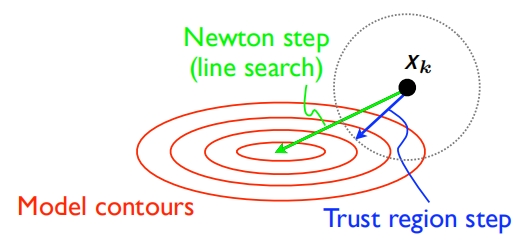信赖域方法的首要为题是如何选择。

定义一个变量衡量信赖域，

分子为实际下降量，分母为预测下降量。

小，说明模型预测的下降量高于模型实际的下降量，需要缩小信赖域。
大，说明模型预测的下降量低于实际模型的下降量，可以采用此信赖域。
 第四章给出了信赖域的算法伪代码，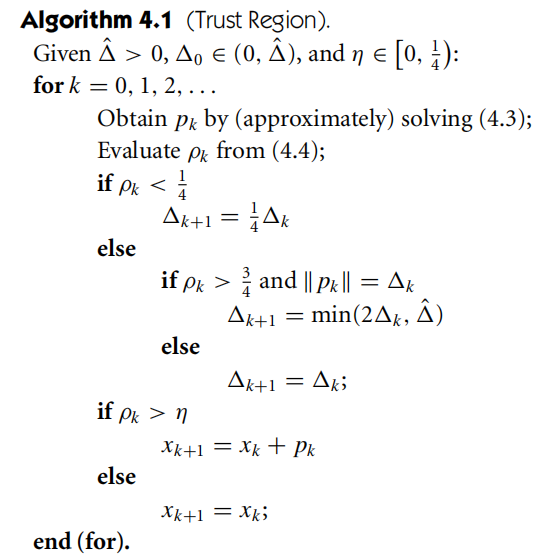典型的信赖度方法有Levenberg-Marquardt，Fletcher的超立方体方法等。下一篇关于最小二乘法的文章中会展开Levenberg-Marquardt法。

下一篇：最小二乘问题。


展开全文梯度下降 最优化
• 标题优化工具包—无约束非线性优化求解器（fminunc） fminunc函数：求无约束多变量函数的最小值 一、fminunc总体介绍 语法： x = fminunc(fun,x0) x = fminunc(fun,x0,options) x = fminunc(problem) [x,fval] = ...matlab
• 无约束非线性优化问题的算法比较研究：包括最常用的几种方法，直接法和解析法
•matlab
• 这个系列将非线性规划是以“不是什么“定义的，也就是说，之前的线性规划模型使用连续决策变量，线性约束和线性目标函数，而非线性规划涵盖了所有其他单目标，...在无约束优化中，线性规划的目标函数无界，而非线性...
• BF共轭梯度法优化无约束非线性问题，求函数极小值，理论和例子参考《最优化方法》（北京理工大学出版社）（程序为自编！！！请不要下架！！！）。压缩包内含matlab程序文件（直接运行BFCG.m），Word文档算例说明....
• 认识面对什么样的实际问题，提出假设和建立优化模型，并且使学生学会使用MATLAB软件进行无约束非线性规划模型求解的基本命令
• 优化原理与方法实验报告： 无约束非线性规划 希望能帮到你
• ©奇异矩 阵和正定矩阵的二次型;(d)不定矩阵的二次型:此时解是一个鞍点，梯度法和 CG 均无法处理该问题。 下降方向 最速下降法也就是梯度法，其中梯度是函数值变化最大的方向。最终通过求导的方式来确定步长。 d=...
• 它被设计为简单，统一的界面，并包装了多个免费/开源非线性优化库。 可以从Github上的页面下载最新版本，并且托管在readthedocs上。 NLopt与构建系统一起编译并安装（有关可用选项，请参阅CMakeLists.txt文件）：...C
• 对励磁系统模型进行了研究,提出了一种新的励磁系统参数优化方法。...对小偏差信号作用下的励磁系统各种暂态响应指标进行比较分析,结果表明所提出的基于约束非线性规划的励磁系统参数优化的方法是有效、可行的。...